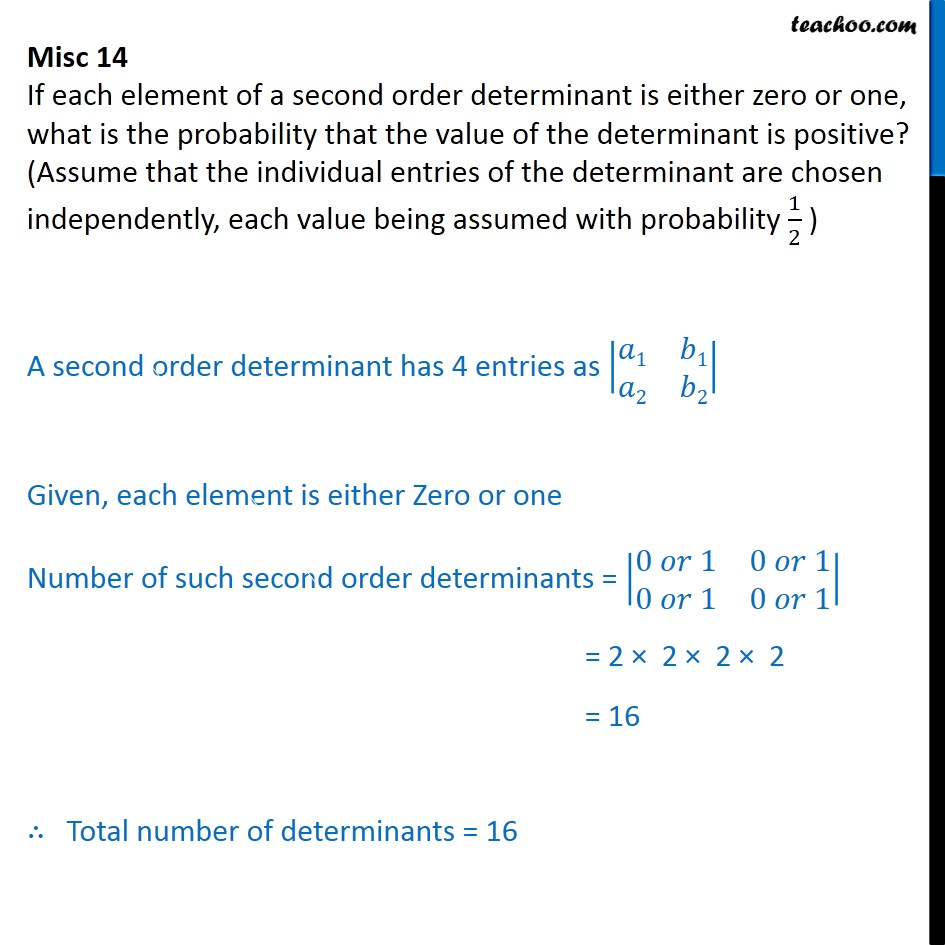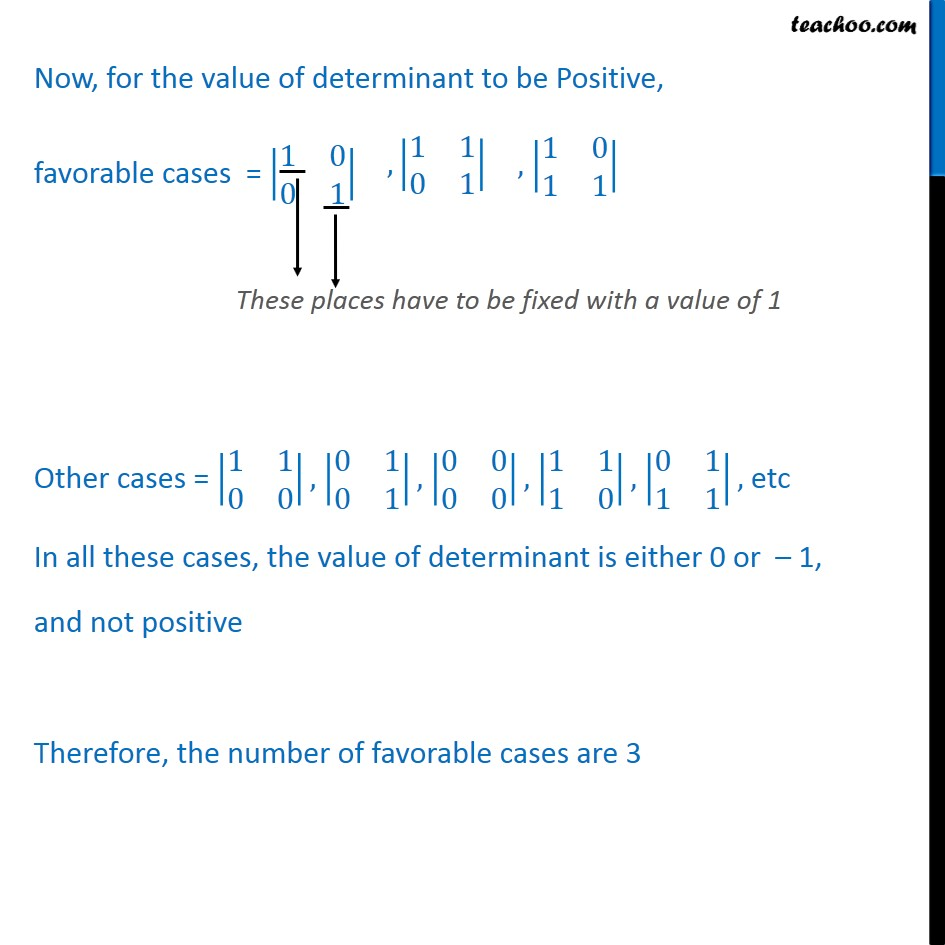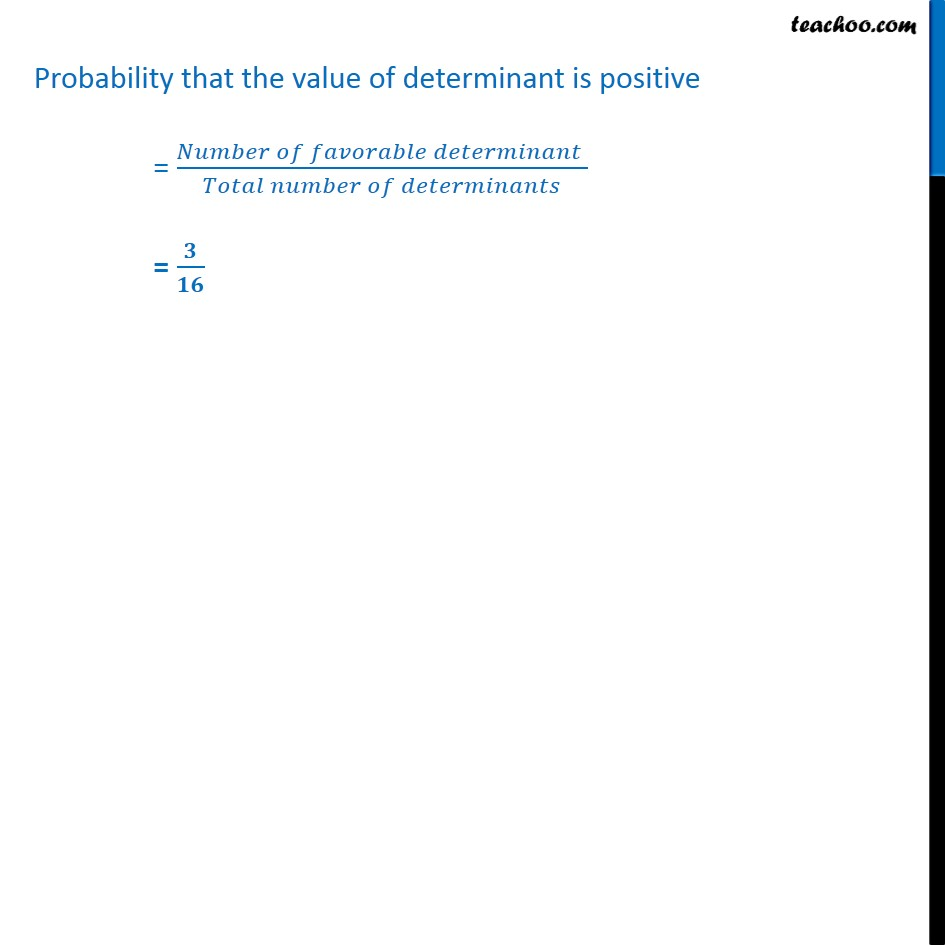1. Chapter 13 Class 12 Probability
2. Serial order wise
3. Miscellaneous

Transcript

Misc 14 If each element of a second order determinant is either zero or one, what is the probability that the value of the determinant is positive? (Assume that the individual entries of the determinant are chosen independently, each value being assumed with probability 1﷮2﷯ ) A second order determinant has 4 entries as 𝑎1﷮𝑏1﷮𝑎2﷮𝑏2﷯﷯ Given, each element is either Zero or one Number of such second order determinants = 0 𝑜𝑟 1﷮0 𝑜𝑟 1﷮0 𝑜𝑟 1﷮0 𝑜𝑟 1﷯﷯ = 2 × 2 × 2 × 2 = 16 ∴ Total number of determinants = 16 Now, for the value of determinant to be Positive, favorable cases = 1﷮0﷮0﷮1﷯﷯ Other cases = 1﷮1﷮0﷮0﷯﷯, 0﷮1﷮0﷮1﷯﷯, 0﷮0﷮0﷮0﷯﷯, 1﷮1﷮1﷮0﷯﷯, 0﷮1﷮1﷮1﷯﷯, etc In all these cases, the value of determinant is either 0 or – 1, and not positive Therefore, the number of favorable cases are 3 Probability that the value of determinant is positive = 𝑁𝑢𝑚𝑏𝑒𝑟 𝑜𝑓 𝑓𝑎𝑣𝑜𝑟𝑎𝑏𝑙𝑒 𝑑𝑒𝑡𝑒𝑟𝑚𝑖𝑛𝑎𝑛𝑡 ﷮𝑇𝑜𝑡𝑎𝑙 𝑛𝑢𝑚𝑏𝑒𝑟 𝑜𝑓 𝑑𝑒𝑡𝑒𝑟𝑚𝑖𝑛𝑎𝑛𝑡𝑠﷯ = 𝟑﷮𝟏𝟔﷯

Miscellaneous